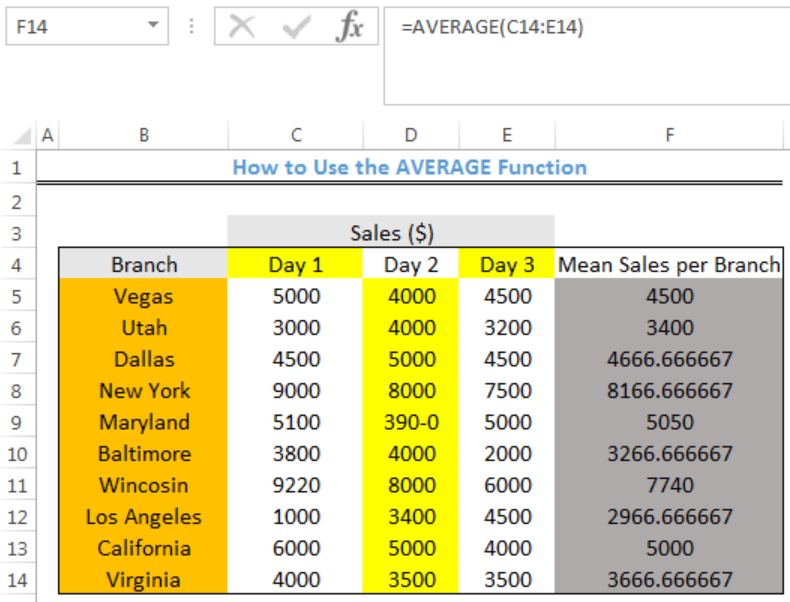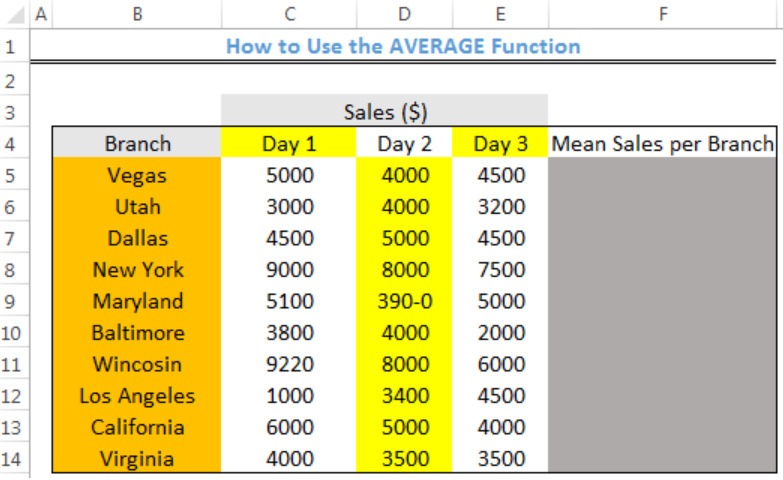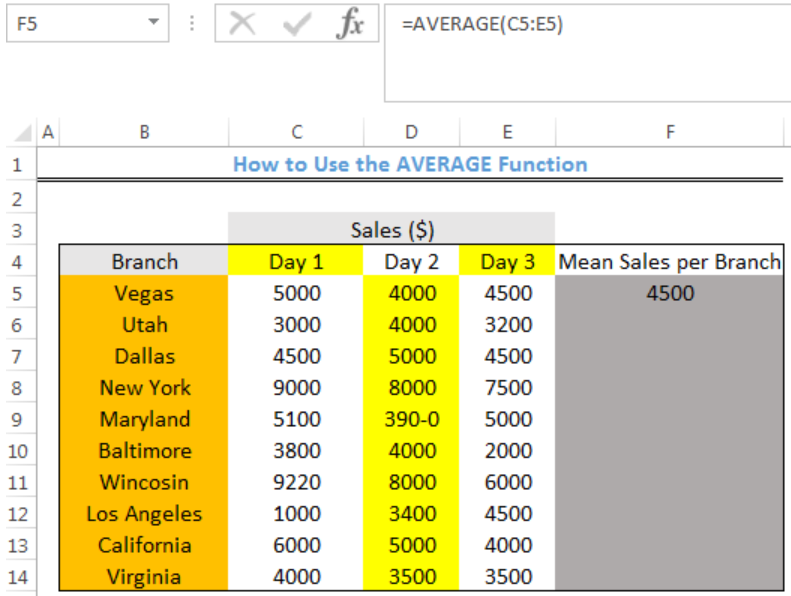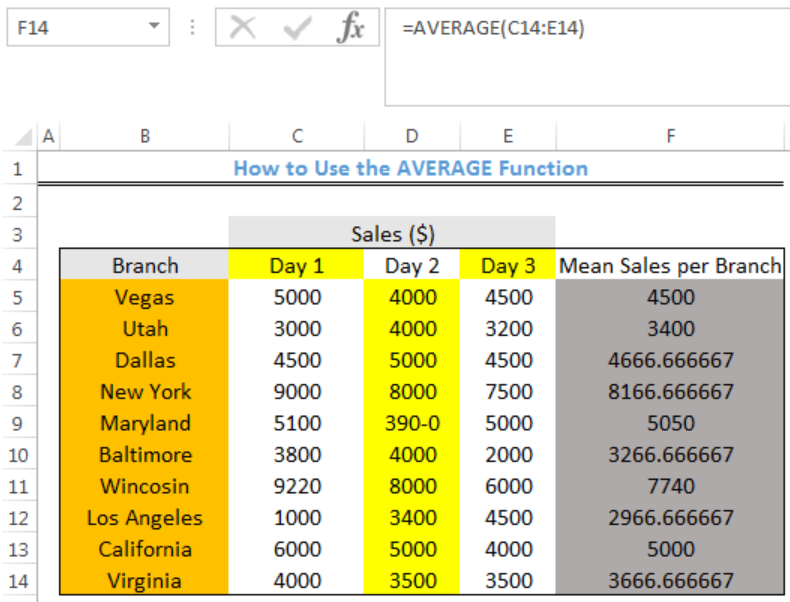Get instant live expert help with Excel or Google Sheets“My Excelchat expert helped me in less than 20 minutes, saving me what would have been 5 hours of work!”

#### Post your problem and you’ll get expert help in seconds.

Your message must be at least 40 characters
Our professional experts are available now. Your privacy is guaranteed.

# Here is How You can Calculate the Mean in Excel

We can use the AVERAGE function to calculate the mean of numbers in a range. The AVERAGE Function can treat up to 255 different arguments. These arguments include numbers, cell references, ranges, arrays, and constants. The steps below will walk through the process.Figure 1- Final result of the AVERAGE function

## Syntax

`=AVERAGE (number1, [number2], ...)`

• number1: This is a number or cell reference that refers to numeric values
• number2 [optional]: This is a number or cell reference that refers to numeric values

## Formula

`=AVERAGE(C5:E5)`

## Setting up the Data

• The Branches of a store will be entered into Column B
• Column C, D, and E will contain the Sales for each of the stores for 3 days
• The mean or average sales for each branch will be returned in Column FFigure 2 – Setting up the Data

## Calculate Mean Sales for each Branch

• We will click on Cell F5
• We will insert the formula below into Cell F5
`=AVERAGE(C5:E5)`
• We will press the enter keyFigure 3- Mean Sales for Vegas

• We will click on Cell F5 again
• We will double click on the fill handle tool which is the small plus sign you see at the bottom right of Cell F5. Select and drag down to copy the formula to other cells.Figure 4- Mean Sales for Stores

## Note

• Any empty cell within the range is ignored by the AVERAGE Function
• The AVERAGE FUNCTION sums the numbers in the range and divides the sum by the total number of numbers

## Instant Connection to an Expert through our Excelchat Service

Most of the time, the problem you will need to solve will be more complex than a simple application of a formula or function. If you want to save hours of research and frustration, try our live Excelchat service! Our Excel Experts are available 24/7 to answer any Excel question you may have. We guarantee a connection within 30 seconds and a customized solution within 20 minutes.

Are you still looking for help with the Average function? View our comprehensive round-up of Average function tutorials here.

Solution examplesOn the Grade Average worksheet, select G7 and type "Average." In cell G8, use a formula to generate the average of the contents in Cells B8:F8. Use the fill handle to populate your results through G10. The syntax for the Excel Average function is the following: AVERAGE(argument1, [argument2],...). Arguments can be numbers, named ranges, cell references that contain numbers, or arrays. Select Cell A12, and enter "Class Average." Use a formula to generate the average of IP Assignment 1 in cell B12. Use the fill across handle to populate your results of averaging the other assignments to F12
Solved by Z. A. in 40 minsI need help calculating average time elapsed. It is in dd:hh:mm:ss text format and so I can't calculate using =AVERAGEIF
Solved by O. E. in 11 minsI contacted you yesterdaya bout how to use the =AVERAGE function, and for real numbers it works fine, but I tried to get the expert to explain to me how to use the =AVERAGE function when the cells have formulas in them that generate '0' value, so the AVERAGE function would ignore these cells in the averaging method, but got no answer. So, I need to know how use the =AVERAGE function so that when there are formulas in the selected cells to average, that EXCEL would ignore those cells like they have real numbers in them, which seems to work fine. Please advise...
Solved by C. J. in 30 minsI need an IF formula where, IF there is a manual adjustment in a whole column of 100% values (say someone changes one to 90%) would say "Yes" or "No". Tried to solve this with =IF(AVERAGE(...)=100%, "No", "Yes") but it is kind of sloppy).
Solved by M. H. in 20 minsIF(AVERAGE(C6+E6+G6)>=5000,TRUE,FALSE) I am using AND function and it is not giving me the correct answer
Solved by G. J. in 24 mins